# Revenue structure of a firm

This page describes some general aspects of the theory of the revenue structure of a firm. For simplicity, we make the following assumptions:

• The firm sells a single type of good.
• The firm establishes a unit price for each sale. We will initially discuss the scenario where the same price is used for all sales by the firm (aka the law of one price). We will later discuss the situation where the firm may practice price discrimination.
• We ignore the issue of heding where firms store some of their produce for later.

## Revenue, price, and quantity

The key formula describing the firm's revenue is: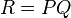$R = PQ$

where$R$ is the revenue,$P$ is the unit price chosen by the firm, and$Q$ is the quantity sold by the firm at that price.

If we consider a graphical representation with quantity sold on the horizontal axis and price of sale on the vertical axis, the revenue is the area of rectangle between the (price, quantity) point and the axes.

### Relation between quantity sold and quantity produced: they're equal for optimal choices

The firm needs to set the price and also decide how much quantity to produce. The quantity it sells is bounded above by the quantity it produces.

To the extent that the firm is capable of predicting the quantity it can sell at a given price, any optimal (price, quantity produced) pair will be such that it sells everything it produces. This is because:

• If the quantity produced is greater than the quantity that can be sold at the given price, the firm wasted resources producing extra stuff.
• If the quantity produced is less than the quantity that can be sold at the given price, the firm can increase its profits by raising the price till the quantity that can be sold falls to the quantity it produces.

### The price function

The price function of a firm is a function that plots, as a function of quantity, the maximum price the firm can choose so that it can sell that quantity at that price. The nature of the price function depends on market structure:

• Monopoly: The price function has the same graph as the market demand curve (note that the role of dependent and independent variable are reversed).
• Perfect competition: The price function is a constant function with value the market price, and the graph is horizontal.

In intermediate situations, such as oligopoly, monopolistic competition, and oligopolistic competition, the price function becomes harder to determine because it depends on the strategic response of other sellers.

### The revenue function

The revenue function plots the firm's total revenue as a function of quantity, assuming that the firm chooses the maximum price at which it can sell that quantity. It is obtained by multiplying the price function by the quantity. Explicitly, if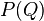$P(Q)$ and$R(Q)$ denote the price and revenue functions, then: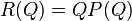$R(Q) = QP(Q)$

### The marginal revenue function

The marginal revenue function is the derivative of the revenue function with respect to quantity. Explicitly:$MR(Q) = \frac{d(R(Q))}{dQ}$

## Revenue: the case of variable pricing or multiple goods

If the firm can practice price discrimination for a single good, or sells multiple goods, the total revenue is the sum of the revenues the firm obtains for each good and price choice. Explicitly, if it sells quantity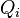$Q_i$ a price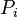$P_i$ for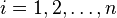$i = 1,2,\dots,n$, then: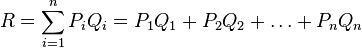$R = \sum_{i=1}^n P_iQ_i = P_1Q_1 + P_2Q_2 + \dots + P_nQ_n$

In case of continuous variation of price and quantity, the revenue may be described as an integral of the form$\int P \, dQ$ or$\int Q \, dP$